# C++对象模型详解

## 何为C++对象模型？

C++对象模型可以概括为以下2部分：

1. 语言中直接支持面向对象程序设计的部分

2. 对于各种支持的底层实现机制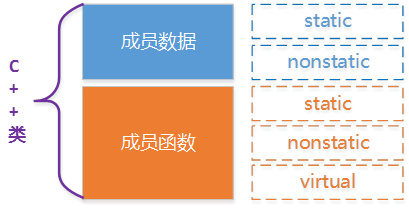```#pragma once
#include<iostream>
using namespace std;
class Base
{
public:
Base(int);
virtual ~Base(void);

int getIBase() const;
static int instanceCount();
virtual void print() const;

protected:

int iBase;
static int count;
};```

Base类在机器中我们如何构建出各种成员数据和成员函数的呢？

## 基本C++对象模型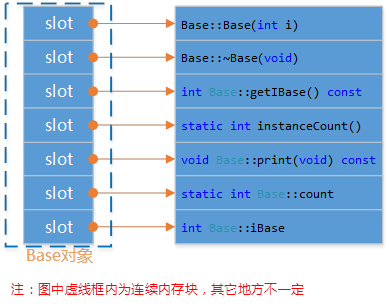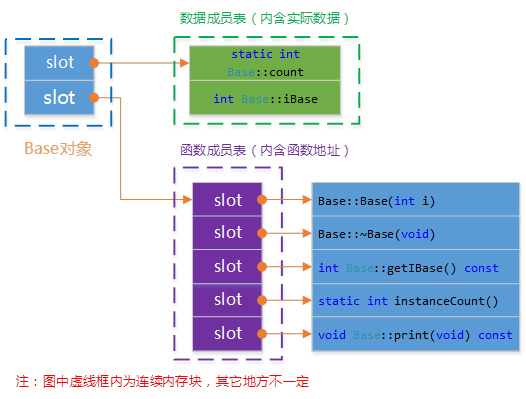C++对象模型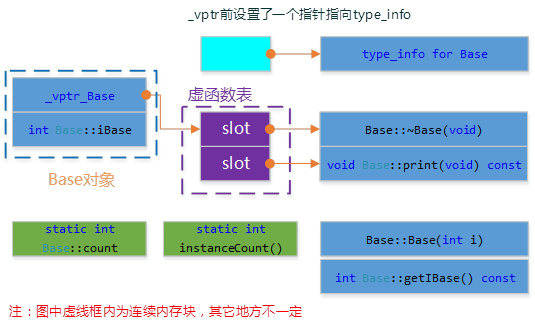1. 每一个class产生一堆指向虚函数的指针，放在表格之中。这个表格称之为虚函数表（virtual table，vtbl）。

2. 每一个对象被添加了一个指针，指向相关的虚函数表vtbl。通常这个指针被称为vptr。vptr的设定（setting）和重置（resetting）都由每一个class的构造函数，析构函数和拷贝赋值运算符自动完成。

```void test_base_model()
{
Base b1(1000);
cout << "对象b1的起始内存地址：" << &b1 << endl;
cout << "type_info信息：" << ((int*)*(int*)(&b1) - 1) << endl;
RTTICompleteObjectLocator str=
*((RTTICompleteObjectLocator*)*((int*)*(int*)(&b1) - 1));
//abstract class name from RTTI
string classname(str.pTypeDescriptor->name);
classname = classname.substr(4,classname.find("@@")-4);
cout << classname <<endl;
cout << "虚函数表地址：\t\t\t" << (int*)(&b1) << endl;
cout << "虚函数表 — 第1个函数地址：\t" << (int*)*(int*)(&b1) << "\t即析构函数地址：" << (int*)*((int*)*(int*)(&b1)) << endl;
cout << "虚函数表 — 第2个函数地址：\t" << ((int*)*(int*)(&b1) + 1) << "\t";
typedef void(*Fun)(void);
Fun pFun = (Fun)*(((int*)*(int*)(&b1)) + 1);
pFun();
b1.print();
cout << endl;
cout << "推测数据成员iBase地址：\t\t" << ((int*)(&b1) +1) << "\t通过地址取值iBase的值：" << *((int*)(&b1) +1) << endl;
cout << "Base::getIBase(): " << b1.getIBase() << endl;

b1.instanceCount();
cout << "静态函数instanceCount地址： " << b1.instanceCount << endl;
}```

• 虚函数表的中第1个函数地址是虚析构函数地址；
• 虚函数表的中第2个函数地址是虚函数print()的地址，通过函数指针可以调用，进行验证；
• 推测数据成员iBase的地址，为虚函数表的地址 + 1，((int*)(&b1) +1)；
• 静态数据成员和静态函数所在内存地址，与对象数据成员和函数成员位段不一样；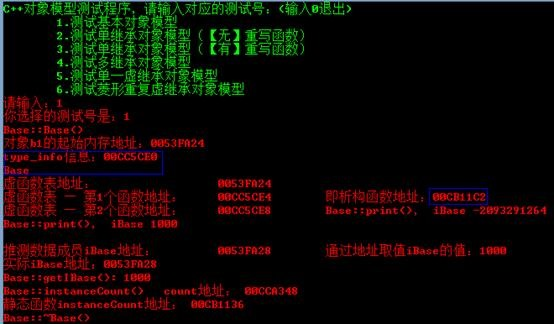## C++对象模型中加入单继承

```#pragma once
#include "base.h"

class Derived :
public Base
{
public:
Derived(int);
virtual ~Derived(void);
virtual void derived_print(void);

protected:
int iDerived;
};```

Base、Derived的类图如下所示：Base的模型跟上面的一样，不受继承的影响。Derived不是虚继承，所以是扩充已存在的虚函数表，所以结构如下图所示：```void test_single_inherit_norewrite()
{
Derived d(9999);
cout << "对象d的起始内存地址：" << &d << endl;
cout << "type_info信息：" << ((int*)*(int*)(&d) - 1) << endl;
RTTICompleteObjectLocator str=
*((RTTICompleteObjectLocator*)*((int*)*(int*)(&d) - 1));
//abstract class name from RTTI
string classname(str.pTypeDescriptor->name);
classname = classname.substr(4,classname.find("@@")-4);
cout << classname <<endl;
cout << "虚函数表地址：\t\t\t" << (int*)(&d) << endl;
cout << "虚函数表 — 第1个函数地址：\t" << (int*)*(int*)(&d) << "\t即析构函数地址" << endl;
cout << "虚函数表 — 第2个函数地址：\t" << ((int*)*(int*)(&d) + 1) << "\t";
typedef void(*Fun)(void);
Fun pFun = (Fun)*(((int*)*(int*)(&d)) + 1);
pFun();
d.print();
cout << endl;

cout << "虚函数表 — 第3个函数地址：\t" << ((int*)*(int*)(&d) + 2) << "\t";
pFun = (Fun)*(((int*)*(int*)(&d)) + 2);
pFun();
d.derived_print();
cout << endl;

cout << "推测数据成员iBase地址：\t\t" << ((int*)(&d) +1) << "\t通过地址取得的值：" << *((int*)(&d) +1) << endl;
cout << "推测数据成员iDerived地址：\t" << ((int*)(&d) +2) << "\t通过地址取得的值：" << *((int*)(&d) +2) << endl;
}```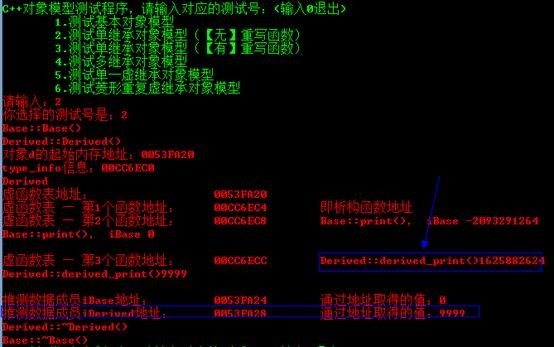```#pragma once
#include "base.h"
class Derived_Overrite :
public Base
{
public:
Derived_Overrite(int);
virtual ~Derived_Overrite(void);
virtual void print(void) const;

protected:
int iDerived;
};```

Base、Derived_Overwrite的类图如下所示：```void test_single_inherit_rewrite()
{
Derived_Overrite d(111111);
cout << "对象d的起始内存地址：\t\t" << &d << endl;
cout << "虚函数表地址：\t\t\t" << (int*)(&d) << endl;
cout << "虚函数表 — 第1个函数地址：\t" << (int*)*(int*)(&d) << "\t即析构函数地址" << endl;
cout << "虚函数表 — 第2个函数地址：\t" << ((int*)*(int*)(&d) + 1) << "\t";
typedef void(*Fun)(void);
Fun pFun = (Fun)*(((int*)*(int*)(&d)) + 1);
pFun();
d.print();
cout << endl;

cout << "虚函数表 — 第3个函数地址：\t" << *((int*)*(int*)(&d) + 2) << "【结束】\t";
cout << endl;

cout << "推测数据成员iBase地址：\t\t" << ((int*)(&d) +1) << "\t通过地址取得的值：" << *((int*)(&d) +1) << endl;
cout << "推测数据成员iDerived地址：\t" << ((int*)(&d) +2) << "\t通过地址取得的值：" << *((int*)(&d) +2) << endl;
}```## C++对象模型中加入多继承

1) 每个基类都有自己的虚表。

2) 子类的成员函数被放到了第一个基类的表中。

3) 内存布局中，其父类布局依次按声明顺序排列。

4) 每个基类的虚表中的print()函数都被overwrite成了子类的print ()。这样做就是为了解决不同的基类类型的指针指向同一个子类实例，而能够调用到实际的函数。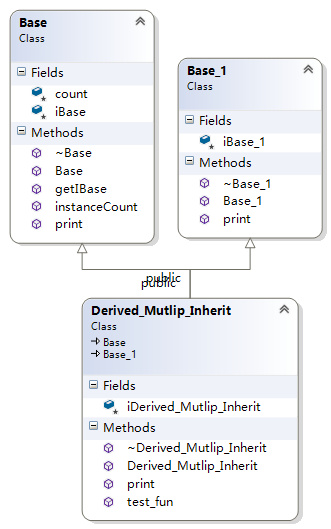```void test_multip_inherit()
{
Derived_Mutlip_Inherit dmi(3333);
cout << "对象dmi的起始内存地址：\t\t" << &dmi << endl;
cout << "虚函数表_vptr_Base地址：\t" << (int*)(&dmi) << endl;
cout << "_vptr_Base — 第1个函数地址：\t" << (int*)*(int*)(&dmi) << "\t即析构函数地址" << endl;
cout << "_vptr_Base — 第2个函数地址：\t" << ((int*)*(int*)(&dmi) + 1) << "\t";
typedef void(*Fun)(void);
Fun pFun = (Fun)*(((int*)*(int*)(&dmi)) + 1);
pFun();
cout << endl;
cout << "_vptr_Base — 第3个函数地址：\t" << ((int*)*(int*)(&dmi) + 2) << "\t";
pFun = (Fun)*(((int*)*(int*)(&dmi)) + 2);
pFun();
cout << endl;
cout << "_vptr_Base — 第4个函数地址：\t" << *((int*)*(int*)(&dmi) + 3) << "【结束】\t";
cout << endl;
cout << "推测数据成员iBase地址：\t\t" << ((int*)(&dmi) +1) << "\t通过地址取得的值：" << *((int*)(&dmi) +1) << endl;

SetConsoleTextAttribute(GetStdHandle(STD_OUTPUT_HANDLE), FOREGROUND_INTENSITY | FOREGROUND_GREEN);
cout << "++++++++++++++++++++++++++++++++++++++++++++++++++++++++++++++" << endl;
SetConsoleTextAttribute(GetStdHandle(STD_OUTPUT_HANDLE), FOREGROUND_INTENSITY | FOREGROUND_RED);
cout << "虚函数表_vptr_Base1地址：\t" << ((int*)(&dmi) +2) << endl;
cout << "_vptr_Base1 — 第1个函数地址：\t" << (int*)*((int*)(&dmi) +2) << "\t即析构函数地址" << endl;
cout << "_vptr_Base1 — 第2个函数地址：\t" << ((int*)*((int*)(&dmi) +2) + 1) << "\t";
typedef void(*Fun)(void);
pFun = (Fun)*((int*)*((int*)(&dmi) +2) + 1);
pFun();
cout << endl;
cout << "_vptr_Base1 — 第3个函数地址：\t" << *((int*)*(int*)((int*)(&dmi) +2) + 2) << "【结束】\t";
cout << endl;
cout << "推测数据成员iBase1地址：\t" << ((int*)(&dmi) +3) << "\t通过地址取得的值：" << *((int*)(&dmi) +3) << endl;
SetConsoleTextAttribute(GetStdHandle(STD_OUTPUT_HANDLE), FOREGROUND_INTENSITY | FOREGROUND_GREEN);
cout << "++++++++++++++++++++++++++++++++++++++++++++++++++++++++++++++" << endl;
SetConsoleTextAttribute(GetStdHandle(STD_OUTPUT_HANDLE), FOREGROUND_INTENSITY | FOREGROUND_RED);
cout << "推测数据成员iDerived地址：\t" << ((int*)(&dmi) +4) << "\t通过地址取得的值：" << *((int*)(&dmi) +4) << endl;
}```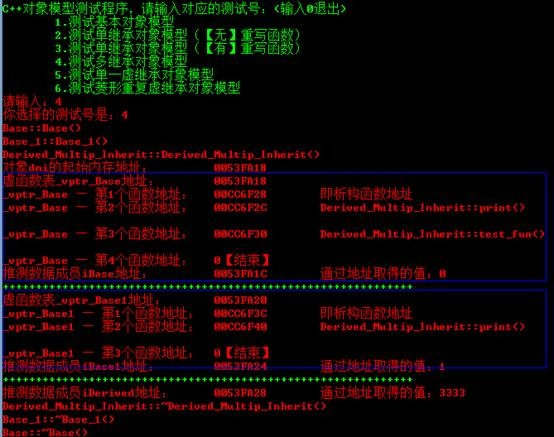## C++对象模型中加入虚继承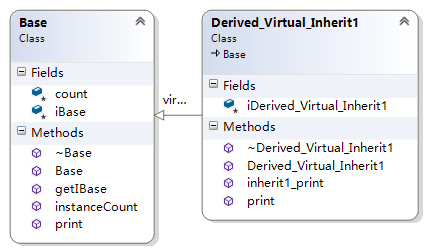Derived_Virtual_Inherit1的对象模型如下图：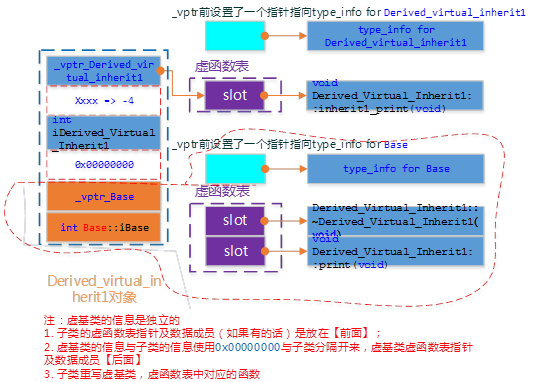```void test_single_vitrual_inherit()
{
Derived_Virtual_Inherit1 dvi1(88888);
cout << "对象dvi1的起始内存地址：\t\t" << &dvi1 << endl;
cout << "虚函数表_vptr_Derived..地址：\t\t" << (int*)(&dvi1) << endl;
cout << "_vptr_Derived — 第1个函数地址：\t" << (int*)*(int*)(&dvi1) << endl;
typedef void(*Fun)(void);
Fun pFun = (Fun)*((int*)*(int*)(&dvi1));
pFun();
cout << endl;
cout << "_vptr_Derived — 第2个函数地址：\t" << *((int*)*(int*)(&dvi1) + 1) << "【结束】\t";
cout << endl;
cout << "=======================：\t" << ((int*)(&dvi1) +1) << "\t通过地址取得的值：" << (int*)*((int*)(&dvi1) +1) << "\t" <<*(int*)*((int*)(&dvi1) +1) << endl;
cout << "推测数据成员iDerived地址：\t" << ((int*)(&dvi1) +2) << "\t通过地址取得的值：" << *((int*)(&dvi1) +2) << endl;
cout << "=======================：\t" << ((int*)(&dvi1) +3) << "\t通过地址取得的值：" << *((int*)(&dvi1) +3) << endl;
cout << "虚函数表_vptr_Base地址：\t" << ((int*)(&dvi1) +4) << endl;
cout << "_vptr_Base — 第1个函数地址：\t" << (int*)*((int*)(&dvi1) +4) << "\t即析构函数地址" << endl;
cout << "_vptr_Base — 第2个函数地址：\t" << ((int*)*((int*)(&dvi1) +4) +1) << "\t";
pFun = (Fun)*((int*)*((int*)(&dvi1) +4) +1);
pFun();
cout << endl;
cout << "_vptr_Base — 第3个函数地址：\t" << ((int*)*((int*)(&dvi1) +4) +2) << "【结束】\t" << *((int*)*((int*)(&dvi1) +4) +2);
cout << endl;
cout << "推测数据成员iBase地址：\t\t" << ((int*)(&dvi1) +5) << "\t通过地址取得的值：" << *((int*)(&dvi1) +5) << endl;
}```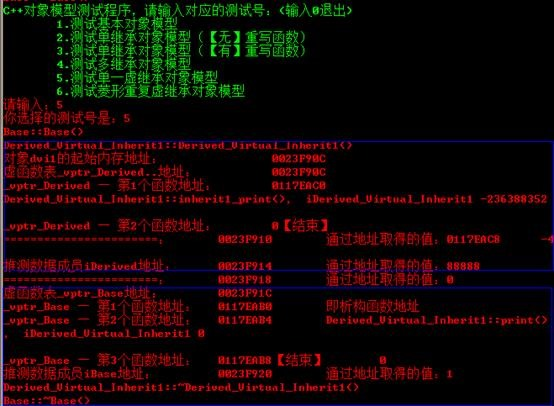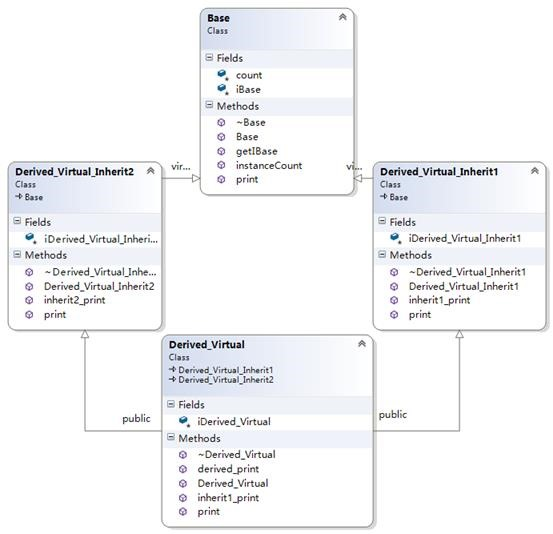Derived_Virtual的对象模型如下图：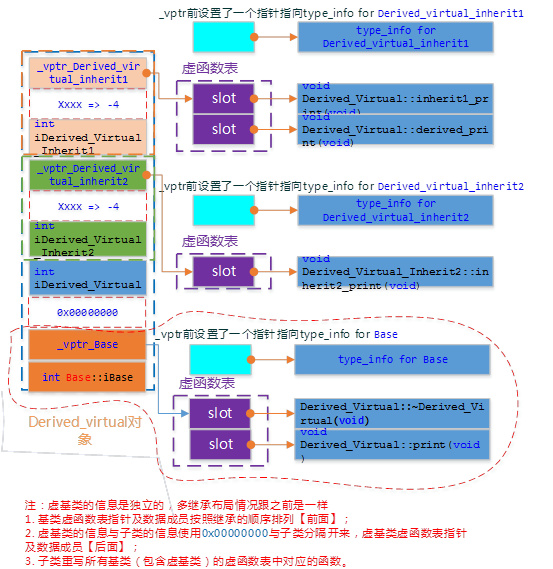```void test_multip_vitrual_inherit()
{
Derived_Virtual dvi1(88888);
cout << "对象dvi1的起始内存地址：\t\t" << &dvi1 << endl;
cout << "虚函数表_vptr_inherit1地址：\t\t" << (int*)(&dvi1) << endl;
cout << "_vptr_inherit1 — 第1个函数地址：\t" << (int*)*(int*)(&dvi1) << endl;
typedef void(*Fun)(void);
Fun pFun = (Fun)*((int*)*(int*)(&dvi1));
pFun();
cout << endl;
cout << "_vptr_inherit1 — 第2个函数地址：\t" << ((int*)*(int*)(&dvi1) + 1) << endl;
pFun = (Fun)*((int*)*(int*)(&dvi1) + 1);
pFun();
cout << endl;
cout << "_vptr_inherit1 — 第3个函数地址：\t" << ((int*)*(int*)(&dvi1) + 2) << "\t通过地址取得的值：" << *((int*)*(int*)(&dvi1) + 2) << "【结束】\t";
cout << endl;
cout << "======指向=============：\t" << ((int*)(&dvi1) +1) << "\t通过地址取得的值：" << (int*)*((int*)(&dvi1) +1)<< "\t" <<*(int*)*((int*)(&dvi1) +1) << endl;
cout << "推测数据成员iInherit1地址：\t" << ((int*)(&dvi1) +2) << "\t通过地址取得的值：" << *((int*)(&dvi1) +2) << endl;
//
cout << "虚函数表_vptr_inherit2地址：\t" << ((int*)(&dvi1) +3) << endl;
cout << "_vptr_inherit2 — 第1个函数地址：\t" << (int*)*((int*)(&dvi1) +3) << endl;
pFun = (Fun)*((int*)*((int*)(&dvi1) +3));
pFun();
cout << endl;
cout << "_vptr_inherit2 — 第2个函数地址：\t" << (int*)*((int*)(&dvi1) +3) + 1 <<"\t通过地址取得的值：" << *((int*)*((int*)(&dvi1) +3) + 1) << "【结束】\t" << endl;
cout << endl;
cout << "======指向=============：\t" << ((int*)(&dvi1) +4) << "\t通过地址取得的值：" << (int*)*((int*)(&dvi1) +4) << "\t" <<*(int*)*((int*)(&dvi1) +4)<< endl;
cout << "推测数据成员iInherit2地址：\t" << ((int*)(&dvi1) +5) << "\t通过地址取得的值：" << *((int*)(&dvi1) +5) << endl;
cout << "推测数据成员iDerived地址：\t" << ((int*)(&dvi1) +6) << "\t通过地址取得的值：" << *((int*)(&dvi1) +6) << endl;
cout << "=======================：\t" << ((int*)(&dvi1) +7) << "\t通过地址取得的值：" << *((int*)(&dvi1) +7) << endl;
//
cout << "虚函数表_vptr_Base地址：\t" << ((int*)(&dvi1) +8) << endl;
cout << "_vptr_Base — 第1个函数地址：\t" << (int*)*((int*)(&dvi1) +8) << "\t即析构函数地址" << endl;
cout << "_vptr_Base — 第2个函数地址：\t" << ((int*)*((int*)(&dvi1) +8) +1) << "\t";
pFun = (Fun)*((int*)*((int*)(&dvi1) +8) +1);
pFun();
cout << endl;
cout << "_vptr_Base — 第3个函数地址：\t" << ((int*)*((int*)(&dvi1) +8) +2) << "【结束】\t" << *((int*)*((int*)(&dvi1) +8) +2);
cout << endl;
cout << "推测数据成员iBase地址：\t\t" << ((int*)(&dvi1) +9) << "\t通过地址取得的值：" << *((int*)(&dvi1) +9) << endl;
}```## 如何访问成员？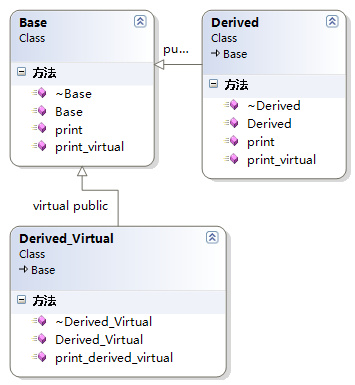• Derived继承Base
• Derived_Virtual虚继承Base
```void test_size()
{
Base b;
Derived d;
Derived_Virtual dv;
cout << "sizeof(b):\t" << sizeof(b) << endl;
cout << "sizeof(d):\t" << sizeof(d) << endl;
cout << "sizeof(dv):\t" << sizeof(dv) << endl;
}```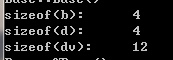```#pragma once
class Empty
{
public:
Empty(void);
~Empty(void);
};```

Empty p，sizeof(p)的大小是多少？事实上并不是空的，它有一个隐晦的1byte，那是被编译器安插进去的一个char。这将使得这个class的两个对象得以在内中有独一无二的地址。

• 每个对象都将增大，增大量为存储虚函数表指针的大小；
• 对于每个类，编译器都创建一个虚函数地址表；
• 对于每个函数调用，都需要执行一项额外的操作，即到虚函数表中查找地址。

## 多态如何实现?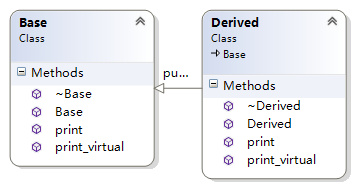• void print() const;
• virtual void print_virtual() const;

```void test_polmorphisn()
{
Base b;
Derived d;

b = d;
b.print();
b.print_virtual();

Base *p;
p = &d;
p->print();
p->print_virtual();
}```

• b.print();b.print_virtual();不能实现多态是因为通过基类对象调用，而非指针或引用所以不能实现多态。
• p->print();不能实现多态是因为，print函数没有声明为虚函数（virtual），派生类中也定义了print函数只是隐藏了基类的print函数。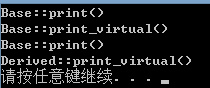```void test_vitual_destructor()
{
Base *p = new Derived();
delete p;
}```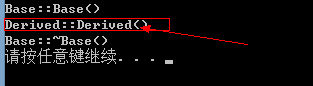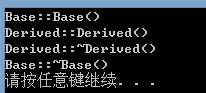(0)
0%

(0)
0%